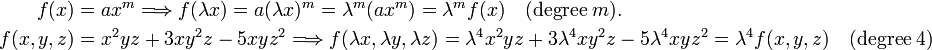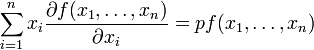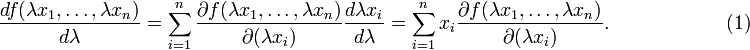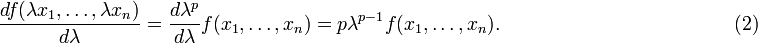# Homogeneous function

In mathematics, a function f,$f: \quad \mathbb{R}^n \rightarrow \mathbb{R}$

is homogeneous of degree p, if$f(\lambda\mathbf{x}) = \lambda^p f(\mathbf{x}),\quad \mathbf{x} \in \mathbb{R}^n, \quad\lambda \in \mathbb{R},\quad p \in \mathbb{N}^*.$

The degree of homogeneity p is a positive integral number.

### Examples\begin{align} f(x) =&\; a x^m \Longrightarrow f(\lambda x) = a (\lambda x)^m = \lambda^m (ax^m) = \lambda^m f(x)\quad(\hbox{degree}\; m). \\ f(x,y,z) =&\; x^2yz + 3 xy^2z -5 xyz^2 \Longrightarrow f(\lambda x, \lambda y, \lambda z) = \lambda^4 x^2yz + 3\lambda^4 xy^2z -5 \lambda^4 xyz^2= \lambda^4 f(x,y,z)\quad(\hbox{degree}\; 4)\\ \end{align}

## Euler's theorem

Let f be differentiable and homogeneous of order p, then$\sum_{i=1}^n x_i \frac{\partial f(x_1, \dots, x_n)}{\partial x_i} = p f(x_1, \dots, x_n)$

### Proof

By the chain rule$\frac{d f(\lambda x_1, \ldots, \lambda x_n)}{d\lambda} = \sum_{i=1}^n \frac{\partial f(\lambda x_1, \dots, \lambda x_n)}{\partial (\lambda x_i)} \frac{d \lambda x_i}{d \lambda} = \sum_{i=1}^n x_i \frac{\partial f(\lambda x_1, \dots, \lambda x_n)}{\partial (\lambda x_i)}. \qquad\qquad\qquad (1)$

From the homogeneity,$\frac{d f(\lambda x_1, \ldots, \lambda x_n)}{d\lambda} = \frac{d\lambda^p}{d\lambda} f(x_1, \dots, x_n) = p \lambda^{p-1} f(x_1, \dots, x_n). \qquad\qquad\qquad \qquad\qquad\qquad(2)$

Compare Eqs (1) and (2) for λ = 1 and the result to be proved follows.